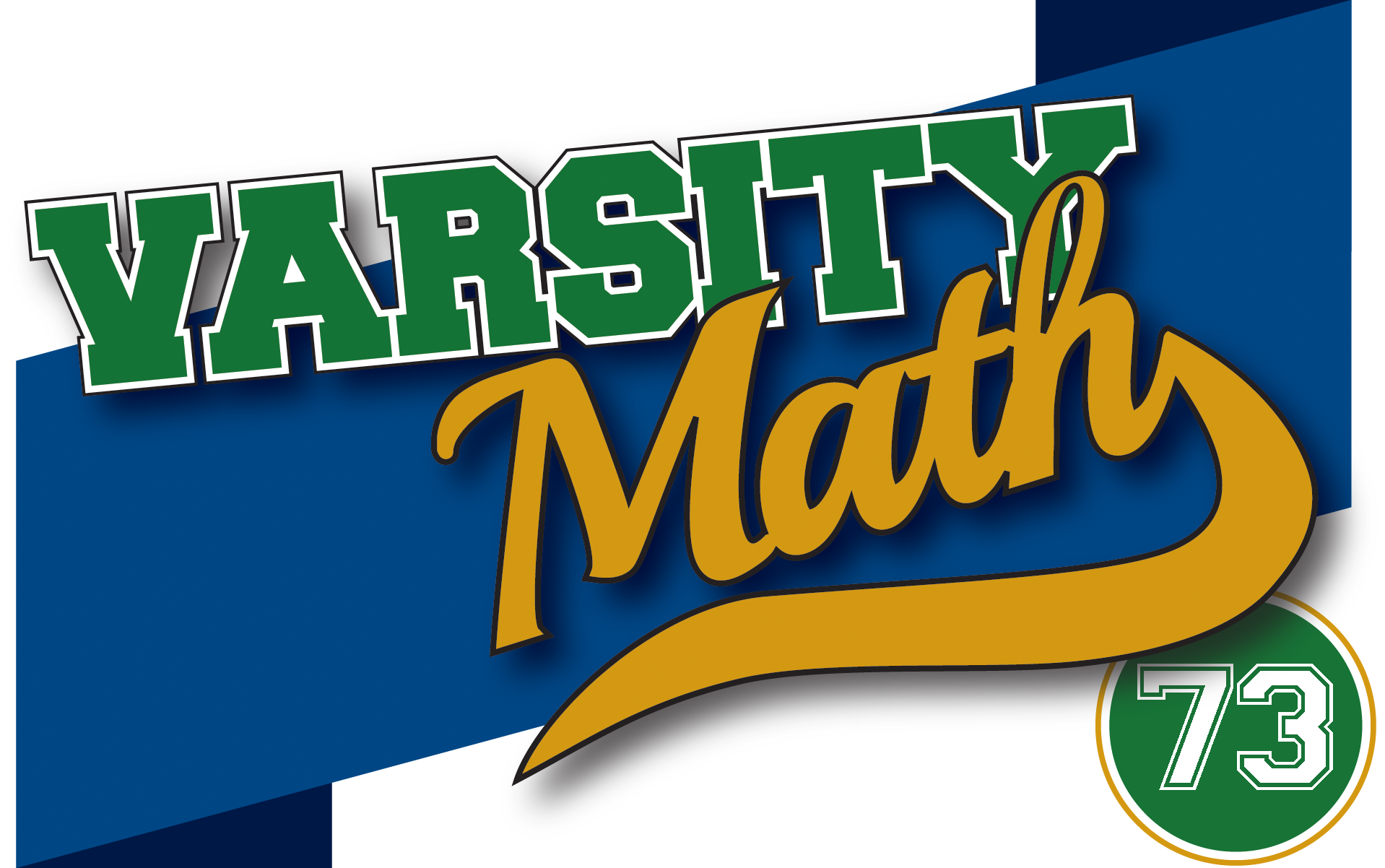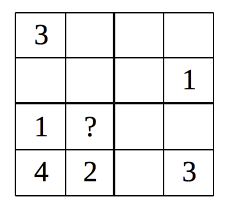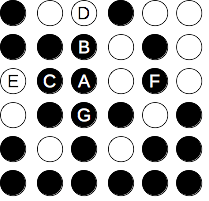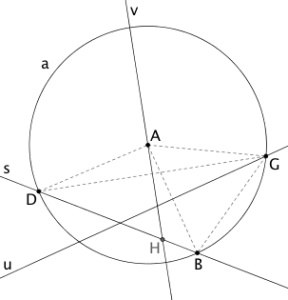## ________________

It’s game night for the Varsity Math team members, but sometime during the evening they become intrigued by what would happen if they played their games on tiny boards.

## ________________

### Small SudokuAriel is solving Sudoku puzzles. Inspired by the evening’s theme, Ariel thinks about 4-by-4 puzzles, in which the solution must contain each of the numbers 1 through 4 in each row, in each column, and in each of the 2-by-2 squares in the corners of the grid. In the example diagram, the space labeled with a “?” must be filled with a three, because the lower left 2-by-2 already has a 1, a 2, and a 4.

What is the smallest number of clues (filled-in squares) that can be given for a 4-by-4 sudoku so that it will have a unique solution? In other words, so that there will only be one way to fill in the rest of the squares while obeying all the rules?

### Puny PegsRaven is playing peg solitaire. Different puzzles have different starting configurations, but all of the pegs are on the grid points of a square grid. On each turn, you can jump any peg over any other adjacent peg horizontally or vertically, removing the peg jumped over.

The diagram shows a 6-by-6 game in progress, where black circles represent pegs and white circles represent empty spaces. Peg A could jump over either peg B or peg C, removing that peg and landing on space D or space E, respectively. It can’t jump over peg F because it isn’t adjacent, and it can’t jump over peg G because the space where it would land is occupied.

What is the smallest square board for peg solitaire in which you can start with every grid point except one occupied and end up with all but one empty?

## Solutions to week 72

The Third Circle.The accompanying diagram shows the givens as described in the problem, with the point of intersection G of lines s and t and some auxiliary line segments added in gray and in dashed lines. Note that since the radii of circles a and b are both AB, triangle ABC is equilateral with all angles equal to 60°. Let x be the measure of angle EBC. Since EC and CF are both radii of the same circle, and segments AF, BE, AC, and BC are all equal, triangles AFC and BEC are congruent. Hence, angle FAC=GAC is also x. Angle ABG is 60-x, and since triangle ADB is isosceles, angle ADG is also equal to 60-x. But now angles ADG, GBA, BAC, and CAG add up to 60-x + 60-x + 60 + x = 180-x, so the only bit of the angles of triangle DAB left, namely angle GAD, must also have measure x to reach the total of 180°. Since triangle DAF is isosceles, angles ADF and DFG must both be 90-x/2. But since ADG is 60-x, this means that angle GDF is 30+x/2. Subtracting the measures of the other two angles of triangle DGF from 180°, we get that the desired angle DGF is 180 – (30+x/2) – (90-x/2) = 60 degrees.

The Second Bisection.Again, we start with a diagram that shows the givens as in the problem, as well as the intersection point H of lines s and v and some auxiliary segments. Since u is the perpendicular bisector of segment AB, GA = GB. Since they are both radii of circle a, GA = AB. Hence, triangle GAB is equilateral, and all of its angles are 60°. Let the measure of angle AGD be y degrees. Then since triangle AGD is isosceles, angle ADG is also y, and angle DAG is 180-2y. Since v is the angle bisector of DAG, angle HAG is 90-y, and subtracting angle BAG=60°, angle HAB is 30-y. On the other hand, angle DBG subtends the long arc DG, which has measure 180+2y, so the measure of angle DBG is 90+y. Subtracting angle GBA, we have angle ABH=30+y. Subtracting both HAB and ABH from 180°, we obtain angle BHA = 120°. Since this is the obtuse angle between lines s and v, we conclude that the acute angle between those lines is also 60 degrees.

## Recent Weeks

Links to all of the puzzles and solutions are on the Complete Varsity Math page.

Come back next week for answers and more puzzles.

[asciimathsf]